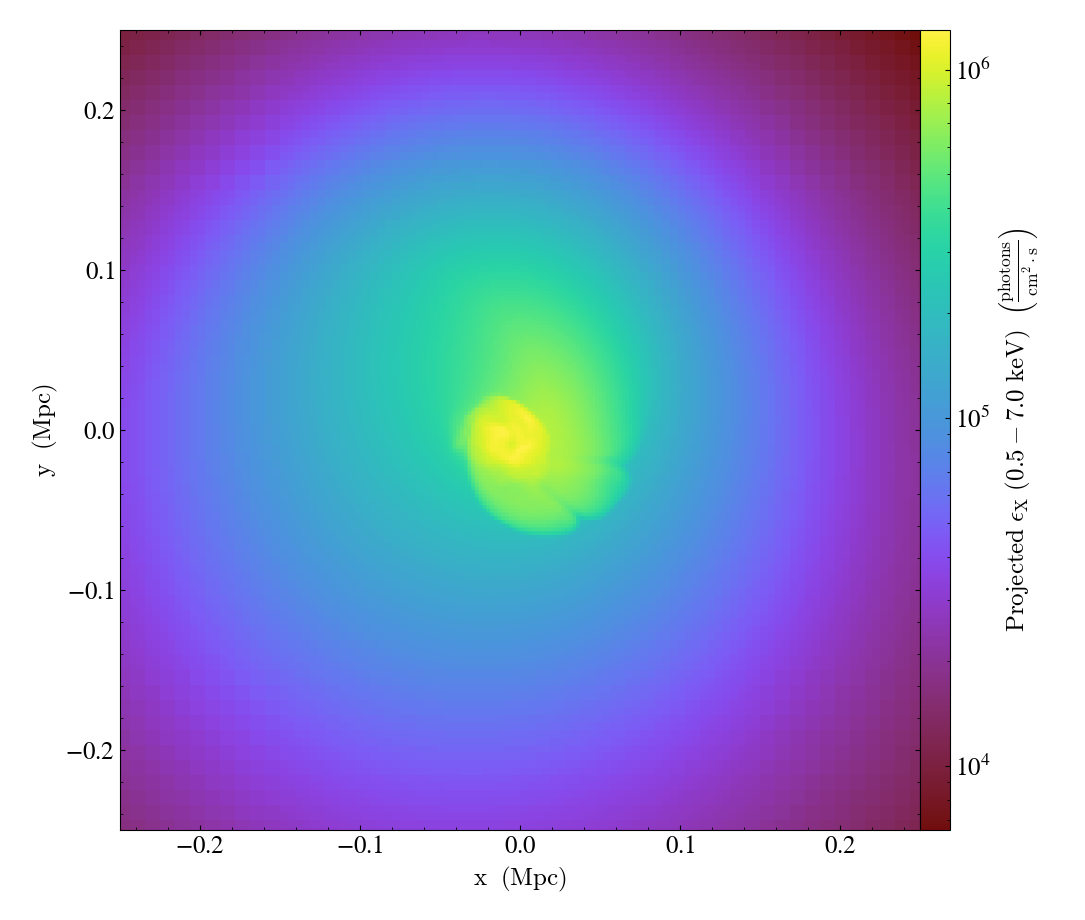# X-ray Fields for yt¶

The simplest use of a SourceModel in pyXSIM is to create fields of X-ray emission that can be used in yt to compute emissivities, luminosities, or intensities. These can be computed for geometric objects such as spheres or boxes or can be projected along a sight line. There are two methods to do this which are described below.

## Source Fields¶

One may want to compute fields for the emissivity or the luminosity of a particular source in its rest frame. This can be done using the make_source_fields() method. This method takes a Dataset object from yt, and the minimum and maximum energies of the band you want to create fields for. The example below shows creating source fields for thermal emission from a simulation of a galaxy cluster:

import yt
import pyxsim

default_species_fields="ionized")

emin = 0.1
emax = 10.0
nbins = 1000
Zmet = 0.3 # this dataset does not have a metallicity field, so assume 0.3 Zsolar
source_model = pyxsim.CIESourceModel("apec", emin, emax, nbins, Zmet)

# arguments are the Dataset, and the emin and emax of the
# band
xray_fields = source_model.make_source_fields(ds, 0.5, 7.0)


The fields are created for the Dataset ds, and their names are returned in the xray_fields list:

print(xray_fields)

[('gas', 'xray_emissivity_0.5_7.0_keV'),
('gas', 'xray_luminosity_0.5_7.0_keV'),
('gas', 'xray_photon_emissivity_0.5_7.0_keV')]


Three fields have been created–one for the X-ray emissivity in the chosen band in $$\rm{erg}~\rm{cm}^{-3}~\rm{s}^{-1}$$, another for the X-ray luminosity in the chosen band in $$\rm{erg}~\rm{s}^{-1}$$, and another for the X-ray photon emissivity in $$\rm{photon}~\rm{cm}^{-3}~\rm{s}^{-1}$$. These fields exist in the same way as any other field in yt, and can be used in the same ways.

Querying emissivity values in a sphere:

sp = ds.sphere("c", (500.0, "kpc"))
print(sp['gas', 'xray_emissivity_0.5_7.0_keV'])

[6.75018212e-30 6.63582106e-30 6.45686636e-30 ... 2.59468150e-30
2.55886161e-30 2.65063999e-30] erg/(cm**3*s)


Summing luminosity in a sphere:

print(sp.sum(("gas", "xray_luminosity_0.5_7.0_keV")))

unyt_quantity(7.73753352e+44, 'erg/s')


Projecting the photon emissivity along a sight line:

prj = yt.ProjectionPlot(ds, "z", xray_fields[-1], width=(0.5, "Mpc"))
prj.save()## Intensity Fields¶

If one wants to compute the fields that are observed locally from the source that is at a given distance or redshift, this can be done using the make_intensity_fields() method. This method takes a Dataset object from yt, the minimum and maximum energies of the band you want to create fields for, and either the cosmological redshift of the source (which gives the distance) or the local distance for a nearby source. These fields are designed specifically for making projections.

The example below shows creating source fields for thermal emission from a simulation of the circumgalactic medium of a disk galaxy:

import yt
import pyxsim

def hot_gas(pfilter, data):
pfilter1 = data[pfilter.filtered_type, "temperature"] > 3.0e5
pfilter2 = data["PartType0", "StarFormationRate"] == 0.0
pfilter3 = data[pfilter.filtered_type, "density"] < 5.0e-25
return pfilter1 & pfilter2 & pfilter3

"hot_gas",
function=hot_gas,
filtered_type="gas",
requires=["temperature", "density"],
)

bounding_box=[[-1000.0, 1000], [-1000.0, 1000], [-1000.0, 1000]])

source_model = pyxsim.IGMSourceModel(
0.2,
3.0,
1000,
("hot_gas", "metallicity"),
binscale="log",
resonant_scattering=False,
cxb_factor=0.5,
kT_max=30.0,
nh_field=("hot_gas","H_nuclei_density"),
temperature_field=("hot_gas", "temperature"),
emission_measure_field=("hot_gas", "emission_measure"),
)

# arguments are the Dataset, the emin and emax of the band, and the redshift
xray_fields = source_model.make_intensity_fields(ds, 0.55, 0.65, redshift=0.01)


The fields are created for the Dataset ds, and their names are returned in the xray_fields list:

print(xray_fields)

[('hot_gas', 'xray_intensity_0.55_0.65_keV'),
('hot_gas', 'xray_photon_intensity_0.55_0.65_keV')]


These can be used to make projections:

prj = yt.OffAxisProjectionPlot(ds, [0.0, -1.0, 1.0], xray_fields[-1],
width=(0.5,"Mpc"), north_vector=[0.0, 1.0, 1.0])
prj.save()Note

At this time, Doppler-shifting of photon energies by motions of the emitting material is not available for the creation of intensity fields in this mode, but it will be available in a future release.